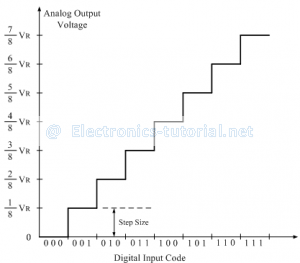Home > analog integrated circuits > data converters > binary weighted resistor dac

# Binary Weighted Resistor DAC

## Binary Weighted Resistor DAC

In the weighted resistor type DAC, each digital level is converted into an equivalent analog voltage or current.
The following figure shows the circuit diagram of the binary weighted resistor type DAC.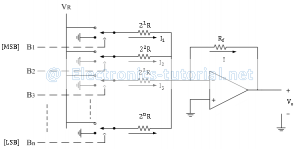It consists of parallel binary weighted resistor bank and a feedback resistor Rf.
The switch positions decides the binary word ( i.e. B1 B2B3…Bn ).
In the circuit op-amp is used as current to voltage converter.

Analysis:
Let us analyze the circuit using normal analysis concepts used in op-amp. When the switches are closed the respective currents are flowing through resistors as shown in the circuit diagram above.
Since input current to the op-amp is zero, the addition current flows through feedback resistor.
∴I=I1+I2+I3+ …………+In
The inverting terminal of op-amp is virtually at ground potential.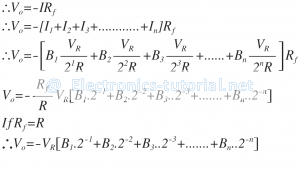Consider the example of 3-bit DAC.
When input binary sequence is B1 B2 B3 = 001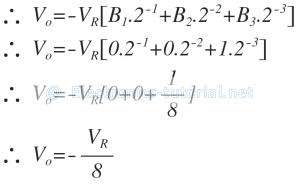When input binary sequence is B1 B2 B3 = 101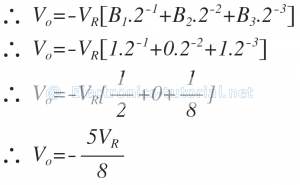If the reference voltage is positive i.e. + VR, then the output voltage is positive.
Similarly for other six combinations of digital input, the analog output voltage Vo is calculated as follows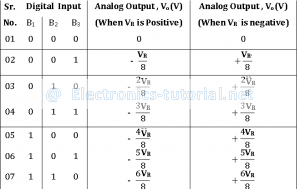The following figure shows the staircase output voltage waveform obtained for R-2R ladder DAC (when VR is positive).